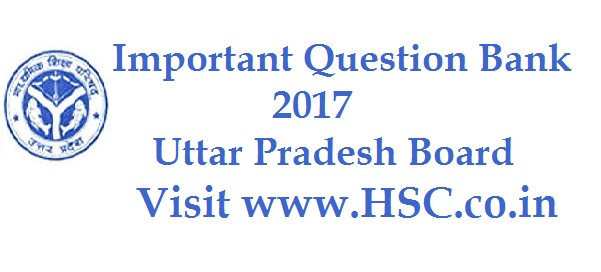# Physics – Important Question Bank for Uttar Pradesh 12th/HSC Board Exam 2017

HSC Board Exams are fast approaching and students are getting anxious about how to prepare for their HSC Board Exams. So we had mentioned some HSC Study Tips to help students in Cracking HSC Exams.

After the tremendous success of our last year Important Questions Bank for Uttar Pradesh Class XII HS (HSC) Board Exam 2016, we have also created a list of Most Important Question Bank for Uttar Pradesh Class XII HS (HSC) Board Exam 2017 which are likely to appear in HSC Board Exams this year.To unlock the content Click on any of 3 buttons available in the box below saying “This content is Locked”. Once you click on the button the content will get unlocked on same page itself. You must click on social media button showing in below box ie Facebook, Twitter or Google Plus to unlock the content.

Hi, we’re trying to collate and gather the data and would be updating it here a few days before the exam. Please keep on visiting our website for updates.

Please use the comments box below and post questions that you think are important from your analysis. It would help the HSC community a lot.

Do subscribe to our updates so that you do not miss out on any important information that we push your way.

1. What is an Oscillator? Draw necessary circuit diagram to show the use of a transistor as an oscillator.
2. What is a rectifier? Draw circuit diagram to explain the use of a P-N junction diode as a full wave rectifier.
3. Describe Weston galvanometer (moving coil) under the following points:

a) Labeled diagram

b) Principle

c) Two merits

4. What is meant by the Electric dipole? Obtain expression for the intensity of electric field at a point in end on position due to an electric dipole.

5. Derive expression for the intensity of magnetic field at appoint in end on position due to a short bar magnet.

6. Describe common base N-P-N transistor as amplifier on the following points:

a) Circuit diagram

b) Working

7. Describe Millikan’s method of determining the chare ‘e’ on an electron under the following headings:

i) Diagram of the apparatus

ii) Derivation of the formula.

8. Describe the experimental method of determining reduction factor of a Tangent galvanometer under the following heads.

i) Formula

ii) Diagram

iii) Observation table

iv) Two precautions

9. Obtain expression for the intensity of magnetic field at a point in broad side on the position due to a short bar magnet

10. What is a capacitor? Obtain an expression for the capacitance of a parallel plate capacitor.

11. Write the difference between Potentiometer and Voltmeter (Any four)

12. Derive the relation between the angel of prism for deviation with dispersion and find resultant deviation.

13. (a) Differentiate between Peltier effect and Joule’s effect of heating.

(b) State the importance of Seebeck’s series.

14. Prove that when two charged conductors are joined, there is a loss of energy. Where does this energy go?

15. Describe the sum and difference method for comparison of magnetic moment of two magnet by a vibration magnetometer on the basis of the following points:

i) Derivation of formula

ii) Observation table

iii) Two precautions

16. Derive the expression for magnification of an astronomical telescope and draw the ray diagram

17. Write and explain Kirchhoff’s law in current electricity.

18. How should be 36 cells each of e.m.f. 1.2 volt and internal resistance 1.0 ohm joined so as to obtain maximum current in an external resistance 4.0 ohm? Calculate this maximum current.

19. A and B are two identical Tangent galvanometers. They have different number of turns in their coils. If they are joined is series and when current flows, a deflection of 60˚ is obtained in the galvanometer A and a deflection of 30˚ in the galvanometer B. calculate the ratio of number of turns in their coils.

20. Explain the theory of Vibration magnetometer. Derive expression for the time period of a magnet in earth’s magnetic field in a vibration magnetometer placed in magnetic meridian.

21. Describe the experiment for determination of focal length of a convex lens by the displacement method on the basis of:

i) Derivation of formula

ii) Ray diagram

iii) Precautions (any two)

22. What is a transistor? Explain the working of PNP transistor with the help of circuit diagram

23. Differentiate between the Alternating current and Direct current (any three)

24. What are Fraunhofer’s lines? What is the cause of their presence in the solar spectrum? Give its uses

25. What is Drift velocity? Establish a relationship between the Drift velocity and the current density.

26. Explain the theory of vibration Magnetometer. Derive expression for the time period of a magnet in earth’s magnetic field in a vibration magnetometer placed in magnetic meridian.

27. A cell is connected between two points on the circumference of a circular conductor. calculate the magnetic field at the centre

28. Draw a labeled diagram of laboratory method for determination of speed of light

29. What is Laser? State its uses (any two)

30. On reversing the telescope, the image becomes too small. why?

Don’t forget to read : MUST REMEMBER THINGS on the day of Exam for HSC Students